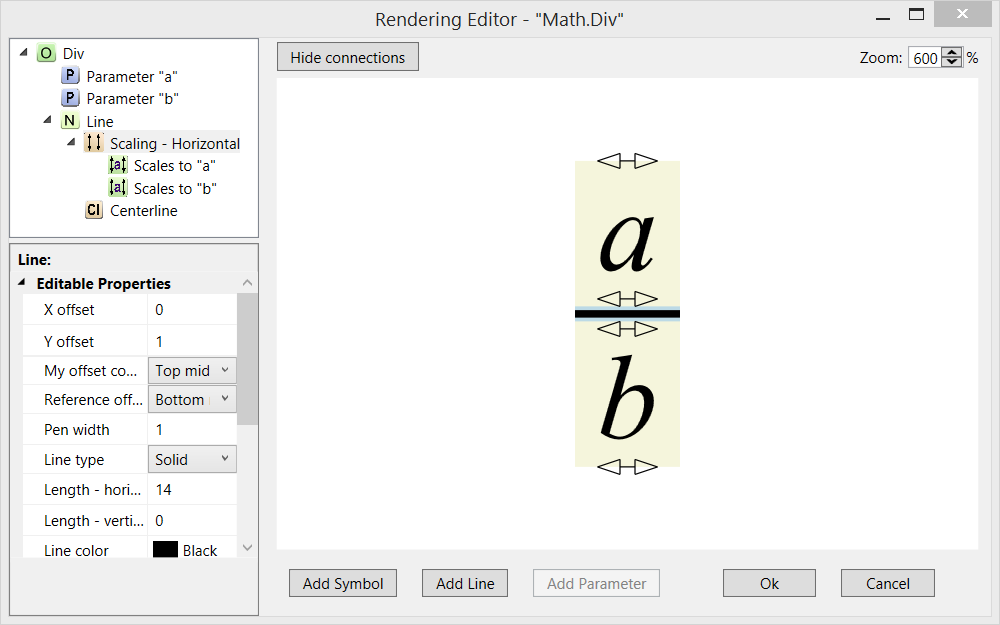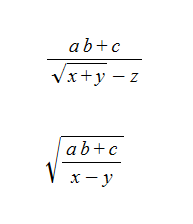# Scaling Symbols and Lines

While some symbols will always stay the same size, the ‘+’ sign for example, others need to scale to the size of the equation. The division line, parentheses, and the square root object are examples. Scaling can only be applied to symbols and lines.

The Math.Div object in the Math.eql library is an example of an object that uses horizontal scaling of a line:Both expressions have the form Div[x, y]. Because the first expression contains single characters in the numerator and denominator, the division line is rendered with its default size. The second expression has larger expressions in both the numerator and denominator so the division line is scaled to the largest expression. A single line or symbol can only scale in one direction. That is, the same line cannot scale both horizontally and vertically in a single object.

If we open the rendering of the Math.Div object in the Rendering Editor, we can see how the line scales horizontally in relation to the a and b parameters. The scaling entries under the line entry in the Rendering Tree show which parameters scale and the direction of the scaling. Note that you have to select one of the scaling nodes in the Rendering Tree to see the graphical representation of the scaling in the Display Area.The square root symbol requires more complex scaling definitions in order to scale horizontally and vertically for longer or more complex equations. The standard Math.Sqrt object provides vertical scaling to the square root symbol:and horizontally to the line above the parameter:You can see how this scaling looks in these expressions: